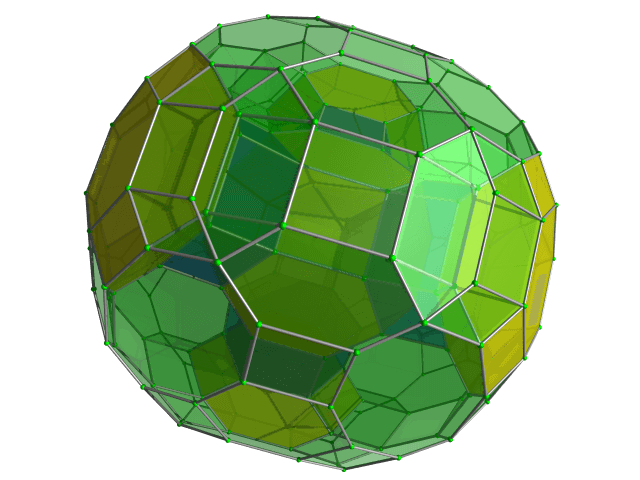Categories

# Mean and Median calculation AMC 8, 2013 Problem 5

Try this beautiful problem from AMC 8. It involves basic statistics and data representation. We provide sequential hints so that you can try the problem.

## Competency in Focus: Mean and Median calculation

This problem from American Mathematics Contest 8 (AMC 8, 2013) is based on calculation of mean and median. It is Question no. 5 of the AMC 8 2013 Problem series.

## First look at the knowledge graph:-## Next understand the problem

Hammie is in the$6^\text{th}$ grade and weighs 106 pounds. His quadruplet sisters are tiny babies and weigh 5, 5, 6, and 8 pounds. Which is greater, the average (mean) weight of these five children or the median weight, and by how many pounds?
##### Source of the problem
American Mathematical Contest 2013, AMC 8 Problem 5

### Basic Statistics and Data Representation mainly calculation of mean and median.

4/10
##### Suggested Book
Challenges and Thrills in Pre College Mathematics Excursion Of Mathematics

Do you really need a hint? Try it first!

Let us first find the median of the weight of the five children. For this, we first have to arrange the weights of the five children in increasing order. As we know, the median is the middle value, if there is an odd number of observations, and if there is an even number of observations, it is the average of the two middle values. Thus, lining up the numbers (5, 5, 6, 8, 106), we see that it  is 6 pounds.

Now what we have to find is the mean of the weights of five children .The average weight of the five kids is$\dfrac{5+5+6+8+106}{5} = \dfrac{130}{5} = 26$.
The median here is obviously less than the mean.
Therefore, the average weight is bigger than median weight , by$26-6 = 20$ pounds, making the answer , average by 20.

## AMC - AIME Program

AMC - AIME - USAMO Boot Camp for brilliant students. Use our exclusive one-on-one plus group class system to prepare for Math Olympiad## External Tangent | AMC 10A, 2018 | Problem 15

Try this beautiful Problem on geometry based on circle from AMC 10A, 2018. Problem-15. You may use sequential hints to solve the problem.

## Dice Problem | AMC 10A, 2014| Problem No 17

Try this beautiful Problem on Probability from AMC 10A, 2014. Problem-17, You may use sequential hints to solve the problem.

## Problem on Curve | AMC 10A, 2018 | Problem 21

Try this beautiful Problem on Co-ordinate geometry from AMC 10A, 2018. Problem-21, You may use sequential hints to solve the problem.

## Right-angled Triangle | AMC 10A, 2018 | Problem No 16

Try this beautiful Problem on triangle from AMC 10A, 2018. Problem-16. You may use sequential hints to solve the problem.

## Finding Greatest Integer | AMC 10A, 2018 | Problem No 14

Try this beautiful Problem on Algebra from AMC 10A, 2018. Problem-14, You may use sequential hints to solve the problem.

## Length of the crease | AMC 10A, 2018 | Problem No 13

Try this beautiful Problem on triangle from AMC 10A, 2018. Problem-13. You may use sequential hints to solve the problem.

## Right-angled shaped field | AMC 10A, 2018 | Problem No 23

Try this beautiful Problem on triangle from AMC 10A, 2018. Problem-23. You may use sequential hints to solve the problem.

## Area of region | AMC 10B, 2016| Problem No 21

Try this beautiful Problem on Geometry on Circle from AMC 10B, 2016. Problem-20. You may use sequential hints to solve the problem.

## Coin Toss Problem | AMC 10A, 2017| Problem No 18

Try this beautiful Problem on Probability from AMC 10A, 2017. Problem-18, You may use sequential hints to solve the problem.

## GCF & Rectangle | AMC 10A, 2016| Problem No 19

Try this beautiful Problem on Geometry on Rectangle from AMC 10A, 2010. Problem-19. You may use sequential hints to solve the problem.

This site uses Akismet to reduce spam. Learn how your comment data is processed.